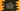# Java Tutorial 1 - Java Math Module## Advanced mathematical computation using Math module in java:

Math class is available in java.lang package and it has two constants and more than 40 static methods to do some advanced mathematical operations easily.

In this tutorial, we will check how to use these methods and what are those constants :

### Constants:

Math module contains two constants :** E** and PI Math.E : It is a double value closer to** ‘e’** , base of natural algorithms Math.PI : It is a double value closer to ‘pi’

### Accessing Math module :

For printing these values and to use methods of Math module we can use static import , which will allow us to access values and methods without writing ‘Math.’ in front of anything . Import statement is look like below :

``import static java.lang.Math.*``

Now let’s print both of these constants :

`````` import static java.lang.Math.*;

public class Test {
public static void main(String[] args){
System.out.println("Math.E = "+E);
System.out.println("Math.PI = "+PI);
}
}``````

It will give us the following outputs :

`````` Math.E = 2.718281828459045
Math.PI = 3.141592653589793``````

### Find absolute value using Math module in java :

To find the absolute value , we can abs() method of Math module. abs method can take double, float, int or long and it returns absolute value of the argument.

`````` import static java.lang.Math.*;

public class Test {

public static void main(String[] args) {
double mDouble = 22.23456E231;
long mLong = 92233720368547758L;
float mFloat = 12.221123432f;
int mInt = 133;

System.out.println("abs of the double " + abs(mDouble));
System.out.println("abs of the long " + abs(mLong));
System.out.println("abs of the float " + abs(mFloat));
System.out.println("abs of the int " + abs(mInt));
}
}``````

#### Output :

`````` abs of the double 2.223456E232
abs of the long 92233720368547758
abs of the float 12.221124
abs of the int 133``````

### Find ceil, floor, rint and double :

ceil, floor and rint takes one double as argument and returns as a double . ceil : return value (double) >= argument floor : return value (double) <= argument rint : returns the int as double which is closest to the argument round : It takes double or float as argument and returns the closest long or int respectively

`````` import static java.lang.Math.*;

public class Test {

public static void main(String[] args) {
double mDouble = 22.24;

System.out.println("ceil of 22.24 " + ceil(mDouble));
System.out.println("floor of 22.24 " + floor(mDouble));
System.out.println("rint of 22.24 " + rint(mDouble));
System.out.println("round of 22.24 " + round(mDouble));

}
}``````

#### Output :

`````` ceil of 22.24 23.0
floor of 22.24 22.0
rint of 22.24 22.0
round of 22.24 22``````

### Find minimum and maximum of two values :

Minimum and maximum of two values can be find using ‘min’ or ‘max’ method of Math module. It takes two arguments to compare and both the arguments should be double,float , int or long.

`````` import static java.lang.Math.*;

public class Test {

public static void main(String[] args) {
double value1 = 22.24;
double value2 = 22.25;

System.out.println("Maximum of value1 and value2 is " + max(value1,value2));
System.out.println("Minimum of value1 and value2 is " + min(value1,value2));

}
}``````

#### Output :

`````` Maximum of value1 and value2 is 22.25
Minimum of value1 and value2 is 22.24``````

### Find exponential, logarithm, power and square root using Math module :

To find exponential, logarithm, power and square root : exp, log , pow and sqrt methods are used respectively . exp, log and sqrt takes one double as argument and returns the result as double. pow takes two doubles : first one is the base and second one is the exponent.

`````` import static java.lang.Math.*;

public class Test {

public static void main(String[] args) {
double value1 = 22.24;
double value2 = 22.25;
double value3 = 4;
double value4 = 2;

System.out.println("Exponential of value1 is " + exp(value1));
System.out.println("logarithm of value2 is " + log(value2));
System.out.println("Square root of value3 is " + sqrt(value1));
System.out.println("Power of value4 as base and value3 as exponent is " + pow(value4, value3));

}
}``````

#### Output :

`````` Exponential of value1 is 4.557317409621067E9
logarithm of value2 is 3.1023420086122493
Square root of value3 is 4.715930449020639
Power of value4 as base and value3 as exponent is 16.0``````

### Trigonometric functions in Math module of Java :

Math module contains some basic trigonometric functions. In the below example, we will check how to find sine, cosine, tangent, arcsine, arccosine, arctangent and how to convert argument to degree or radian. For sine, cosine and tangent, name of the methods used are : sin, cos, tan . Similarly for arc values, asin, acos and atan is used. toDegrees converts an argument to degree and toRadians converts an argument to radians. Each method takes one argument as double.

`````` import static java.lang.Math.*;

public class Test {

public static void main(String[] args) {
double value1 = 30.0;
double value2 = 60.0;
double value3 = 45.0;

System.out.println("Sine of 30.0 is " + sin(toRadians(value1)));
System.out.println("Cosine of 60.0 is " + cos(toRadians(value2)));
System.out.println("Tangent of 45.0 is " + tan(toRadians(value3)));
System.out.println("arcsine of sin(30) is " + toDegrees(asin(sin(toRadians(value1)))));
System.out.println("arccosine of cos(60) is " + toDegrees(acos(cos(toRadians(value2)))));
System.out.println("arctangent of tan(45.0) is " + toDegrees(atan(tan(toRadians(value3)))));

}
}``````

#### Output :

`````` Sine of 30.0 is 0.49999999999999994
Cosine of 60.0 is 0.5000000000000001
Tangent of 45.0 is 0.9999999999999999
arcsine of sin(30) is 29.999999999999996
arccosine of cos(60) is 59.99999999999999
arctangent of tan(45.0) is 45.0``````

### Find random number using random() method :

We can get one random number in the range of 0.0 and 1.0 using Math.random() method. To get a number in different range, simply multiply the output with the top range value. e.g. , to get one random number between 0 and 100, we will have to use the following :

``(int)(Math.random() * 100)``

That’s it . If you have any queries, do leave us a comment below. And, please share this tutorial and website with your friends :) reference :_ oracle doc_